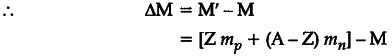# Define the terms (i) mass defect (ii) binding energy for a nucleus and state the relation between the two

Define the terms (i) mass defect (ii) binding energy for a nucleus and state the relation between the two.
For a given nuclear reaction, the B.E. / nucleon of the product nucleus / nuclei is more than that for the original nucleus / nuclei. Is this nuclear reaction exothermic or endothermic in nature ? Justify your choice.

(i) Mass defect \left( \triangle M \right) , of any nucleus _{ Z }^{ A }{ X } is the
difference in the mass of the nucleus (= M) and the sum of masses of its constituent nucleons (= M’).where { m }_{ p } and
{ m }_{ n } denote the mass of the proton and the neutron respectively.
(ii)Binding energy is the energy required to separate a I
nucleus into its constituent nucleons. The relation j between the two is
B.E. = (mass defect) { c }^{ 2 }.
(iii)There is a release of energy, i.e. the reaction is
exothermic.
Reason : Increase in B.E/nucleon implies that the more mass has been converted into energy. This would result in release of energy.